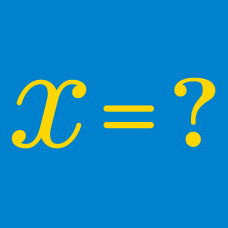Algebra

# Algebra Warmups: Level 5 Challenges

$f(x)=x^{3}-x^{2}-2x+1$

Let the zeros of the function above be $\alpha,\beta,$ and $\gamma.$

Find $f'(\alpha)\times f'(\beta)\times f'(\gamma).$

Note: $f'(x)$ denotes the derivative of $f(x).$

Let $x, y, z\geq 0$ be reals such that $x+y+z=1$. Find the maximum possible value of

$x (x+y)^{2}(y+z)^{3}(x+z)^{4}.$

If a complex number $\alpha$ satisfies the equation $\alpha^3-\alpha^2-2\alpha+1=0,$ where $\alpha=x+\frac{1}{x}$ for some complex number $x$, then what is the value of the expression below? $x^{64}-2x^{52}+3x^{43}+2x^{38}-2x^{29}+5x^{17}+5x^{10}-7x^7+7$

Given 81 variables that satisfy

$0 \leq a_1 \leq a_2 \leq \ldots \leq a_{81} \leq 1,$

what is the maximum value of

$\left[ 9 \sum_{i=1}^{81} a_i ^2 \right] + \left[ \sum_{1 \leq j < k \leq 81 } ( a_k - a_j + 1)^2 \right] ?$

$\begin{array} { l l} a + b + c & \in \mathbb{N} \\ a^4+b^4+c^4 & =32\\ a^5+b^5+c^5& =186\\ a^6+b^6+c^6& =803\\ \end{array}$

What is the value of $a+b+c$?

×